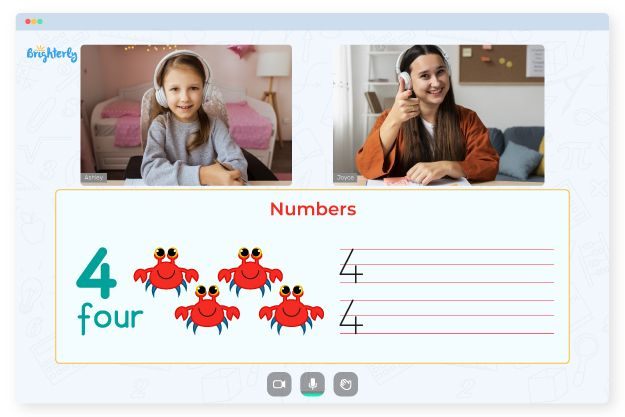# Math Facts Worksheets

Math facts practice worksheets are an essential learning tool for students to practice their math skills. Concepts like addition, subtraction, multiplication, and division are all included in math facts, which are the fundamental building blocks of math. Students must master these abilities because these skills lay the groundwork for more complex math problems.

In this article, we will examine the significance of math fact worksheets, various types of worksheets available, and their effective use.

## Why Are Worksheets on Math Facts Important?

Students must master math facts because this knowledge lays the groundwork for solving more complex math problems. For instance, algebra, trigonometry, and calculus will likely be challenging for a student who struggles with multiplication. Students can practice and improve their math skills by using math fact practice worksheets.

The worksheets’ repetitive nature helps a student retain the concepts and makes it easier to recall the information when needed.

Math facts worksheet also helps students gain confidence in math. It can be demotivating and discouraging for students to experience math difficulties. However, worksheets can boost students’ confidence and motivate them to continue working on their math skills when they see improvement of their skills through practice.

## Worksheets on Math Facts

There are several different worksheets on math facts, and each of them aims to help students improve a particular mathematical skill. The most typical types of math facts worksheets are as follows:

Worksheets on addition are designed to help students get better at adding numbers together. Another essential tool that teachers use to help students improve their mathematical abilities is the addition worksheet.

• ### Multiplication Worksheets

Worksheets on multiplication are another essential part of a student’s math education. Students will learn how to multiply numbers together using these worksheets.

• ### Division Worksheets

Another essential tool for teaching math to students is division worksheets. Students learn how to divide numbers with the assistance of these worksheets.

• ### Mixed Operation Worksheets

Students can use mixed operation worksheets to practice their math skills in various subjects. Thanks to the worksheets that combine many different math operations students can put their knowledge to use in various situations.

Math for Kids

Is Your Child Struggling With Math?
1:1 Online Math Tutoring## How to Use Math Facts Worksheets Effectively

Using basic math facts worksheets effectively is crucial to get the most out of them. The ways to use math facts worksheets effectively are as follows:

When introducing a new idea, start with easy problems and work up to more difficult ones. Students benefit from this by gaining self-assurance and avoiding frustration.

• ### Use real-world examples

Use real-world examples whenever possible to help students comprehend the significance of math concepts. For example, when teaching addition, use activities like counting money or adding items in a grocery store.

• ### Encourage students to display their work when solving problems

Encouraging students to show their work, educators and parents are able to identify potential areas of difficulties and provide additional assistance.

• ### Constructive criticism

Give students constructive criticism of their performance. It motivates them to keep practicing and helps them comprehend where to improve.

## Benefits

Worksheets give students a chance to practice their math skills. As a result, students are more likely to retain the material and benefit from the concepts being reinforced through repetition.

Secondly, students are free to work at their own pace. Taking time to complete each worksheet helps students feel less anxious and stressed.

Thirdly, worksheets aid in boosting students’ math confidence. Students gain confidence in solving math problems as they become more familiar with the concepts.

Teachers and parents alike can benefit from the free math facts worksheets. They allow teachers to see how well their students understand math concepts. Teachers can use the worksheets to pinpoint areas where students require additional assistance and modify lessons accordingly.

Parents can also use the worksheets to assist their children with homework or provide additional practice outside school.### Math Facts Worksheets PDF

Math Fact Practice Worksheets### Math Facts Worksheets PDF

Math Fact Worksheets### Math Facts Worksheets PDF

Math Facts Practice Worksheets### Math Facts Worksheets PDF

Math Facts Worksheet

Need help with Numbers?• Is your child struggling to master numbers?
• Consider learning with an online tutor.

Does your child find the concept of numbers difficult? An online tutor could provide the necessary assistance.